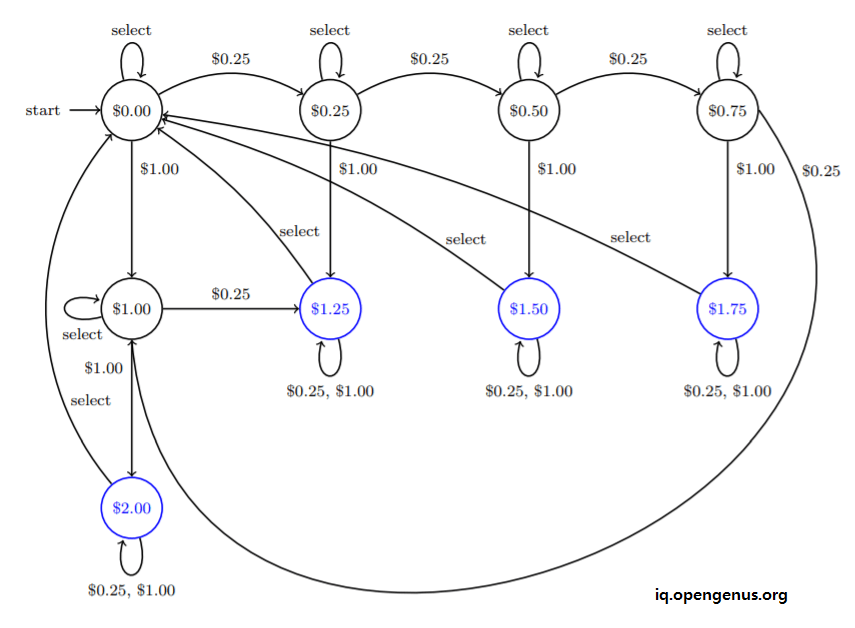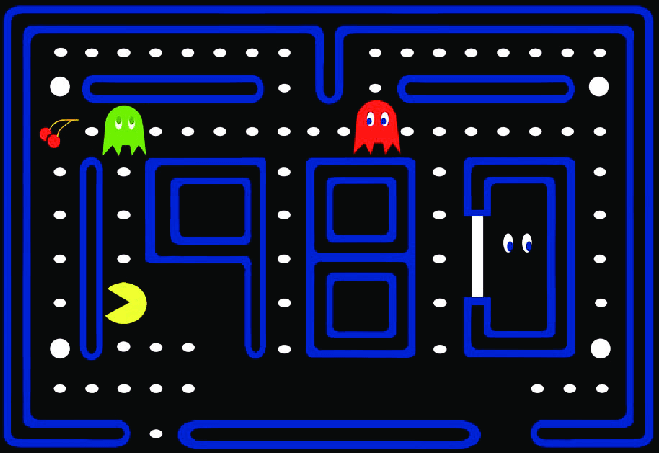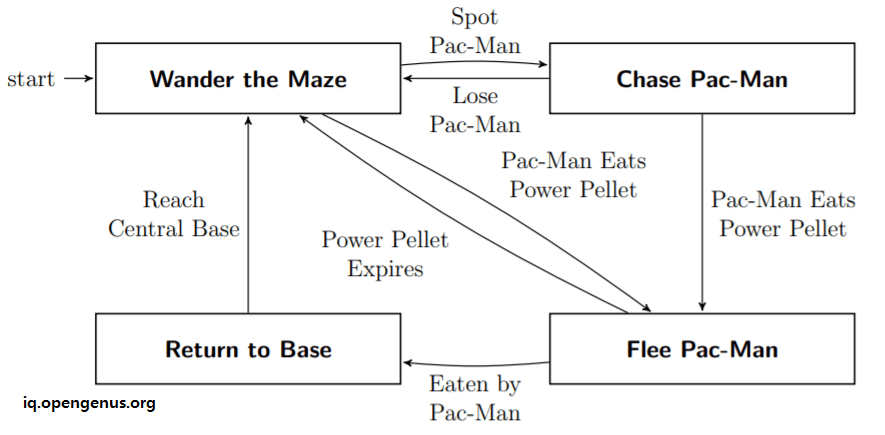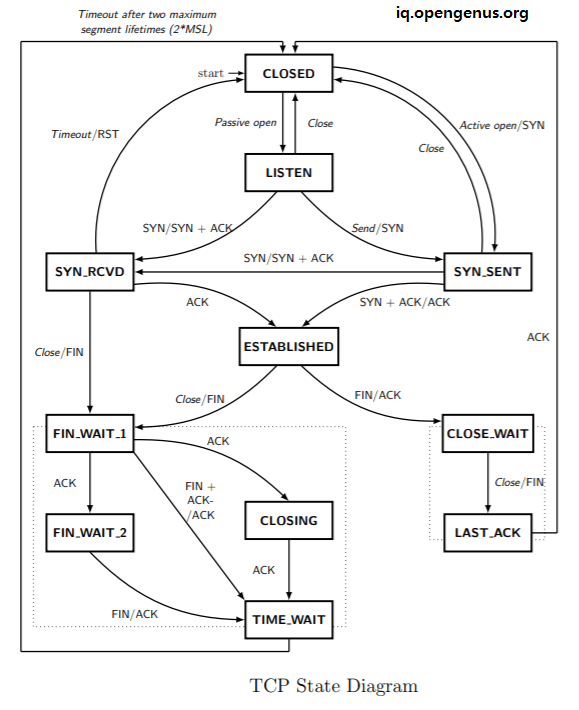# Applications of Theory of ComputationIn this article, we have listed and explained the most important applications of ideas in Theory of Computation that involves DFA, Turing Machine, Definition of Algorithms and much more. This is a must read to understand the importance of Theory of Computation.

Applications of Theory of Computation are:

• Game of Life: The most popular application of merging of Automata Theory (Theory of Computation) and Biology
• Complexities in Natural Selection in Biology can be modeled using Automata Theory (Theory of Computation)
• Entire Universe can be modeled with a Automata Machine in Theory of Computation and is similar to existing theories in Physics
• Deterministic Finite Automata (DFA) is used in Compiler Design where the first step is lexer (lexical analysis)
• Models in Theory of Computation are used to model real life Computing Machines and Problems.
• Models in Theory of Computation can be used to find limitation of Computing Machines like Halting Problem.
• Super Recursive Algorithms in Theory of Computation present the future of Computing Devices.
• Other real life applications of DFA:
• Traffic Lights
• Video Games
• CPU Controllers
• Protocol Analysis
• Regular Expression Matching
• Vending Machines
• Speech Recognition
• Natural Language Processing
• Auto-complete search in Apache Lucene uses Finite State Transducer (FST) (extension of DFA)
• DFAs for simple Artificial Intelligence applications.
• DFAs with probabilistic transitions are known as Markov Chain.
• All Algorithms are designed using Turing Machine.

Now, you know that Theory of Computation is very useful in real life and the ideas you learn in Theory of Computation can be applied widely.

We will now dive further into some of the applications of Theory of Computation.

# Vending Machines using ToC

Vending Machines are machines installed in a city on the side of roads at specific intervals. Citizens can insert money into these machines and get the selected drinks and snacks automatically and the return change was provided by the machine as well. Such machines are very popular in Japan.

The working of Vending Machine can be designed using Deterministic Finite Automata (DFA) in ToC.

The states of Deterministic Finite Automata (DFA) for Vending Machines include:

• Q = {\$0.00, \$0.25, \$0.50, \$0.75, \$1.00, \$1.25, \$1.50, \$1.75, \$2.00} (states)
• Σ = {\$0.25, \$1.00, select} is the alphabet
• q0 = \$0.00 is the start state
• F = ∅ is the set of accept states

The transition function is defined by the state diagram.

The state diagram of Vending Machine is as follows:# Pac Man game using ToC

Pac Man is a popular game in the early 1990s. Modeling the game involves ideas of DFA (Deterministic Finite Automata). Similar designing all games involve DFA so that you can create a games with a large number of possible levels and situation starting for a few set of rules and stating states.

Following is a screenshot of the Pac Man game:Following is the State Diagram of a DFA for Pac Man Game:# TCP using Automata Machine

Transmission Control Protocol (TCP) is the basis of Computer Networking and the possible states and situations are designed using Automata Machine.

Following is the state diagram of a DFA for TCP:# Algorithms = Turing Machine

In Computer Science, the formal definition of Algorithm is that any set of steps that can be performed by a Turing Machine. Several researchers came up with alternative definitions but all boiled down to the same definition using Turing Machine.

Thus, Turing Machine is the fundamental concept in Computer Science and hence, all solutions that can be defined using an Algorithm is using the ideas of Turing Machine and other Computing models from Theory of Computation.

This model of Computing from Theory of Computation is also important to understand computing in general. It proves that the classical Computers are same as Quantum Computers and Molecular Computers. Therefore, Quantum Computers cannot solve a Problem that a Tradition computer cannot solve. The only advantage of Quantum Computer is that it is more efficient than Traditional Computers.

Moreover, Theory of Computer presents Super Recursive Algorithm which is more powerful than the current models like Quantum Computers and Traditional Computers.

# Regular Expressions

Regular Expression is converted to Non-Deterministic Finite Automata (NFA) which is computed easily to check if a given string is accepted by the DFA and hence, the string is accepted by the regular expressions.

Some may try to parse HTML code using Regular Expression but this is not possible and is proved using Pumping Lemma of Theory of Computation.

With this article at OpenGenus, you must have a strong idea of how ideas in Theory of Computation are applied in real life applications. Therefore, Theory of Computation is one of the most important domains in Computer Science.